# Digital Comparator

Suppose we have two binary numbers which we have to compare according to their magnitude. One number of these two numbers can either be greater, equal or smaller than the other number. The digital circuit which performs this comparison task between binary numbers is called digital comparator. For understanding better let us consider two single bit binary numbers A and B. The value of A and B either be 0 or 1 and nothing else. Now let us logically design a circuit which will have two inputs one for A and other for B and have three output terminals, one for A > B condition, one for A = B condition and one for A < B condition. Let us name the output terminals G, E and L respectively.

We want,
G = 1 (logically 1) when A > B.
B = 1 (logically 1) when A = B.
And
L = 1 (logically 1) when A < B.
If we successfully design this logic circuit, it will confidently compare two single bit binary numbers A, B and gives high state at respective output terminal according to the comparison conditions of A and B.

 A B G E L 0 0 0 1 0 0 1 0 0 1 1 0 1 0 0 1 1 0 1 0

When, A = 0 and B = 0, then A = B and E = 1
When, A = 0 and B = 1, then A < B and L = 1
When, A = 1 and B = 0, then A > B and G = 1
When, A = 1 and B = 1, then A = B and E = 1

Now from above table, we get,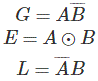This circuit can be realized as,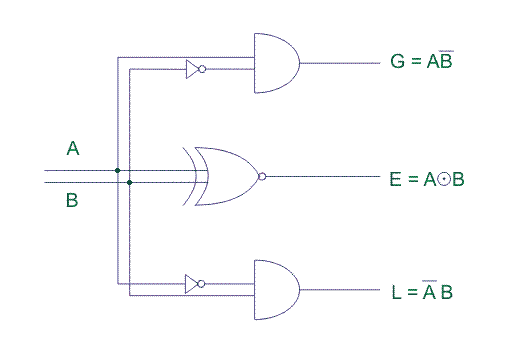As the above can only compare two single bit binary numbers, it is called single bit digital comparator.
The binary number system normally does not use single binary numbers instead it uses multi bit binary numbers which are normally 4 bits and above. So, let us design a 4 bit digital comparator to get more clear idea of comparator.
Suppose, there are two 4 bit binary numbers,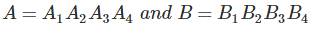Let us compare those two numbers
Condition (1), when A1 > B1 i.e. A1 = 1 and B1 = 0, ⇒ A > B or G = 1.
Condition (2), when A1 = B1 and A2 > B2 i.e. A2 = 1 and B2 = 0 ⇒ A >B or G = 1.

Condition (3), when A1 = B1 and A2 = B2 and A3 > B3 i.e. A3 = 1 and B3 = 0 ⇒ A >B or G = 1.
Condition (4), when A1 = B1, A2 = B2, A3 = B3 and A4 > B4 i.e. A4 = 1 and B4 = 0 ⇒ A > B or G = 1.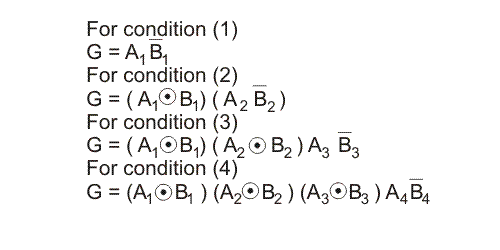Hence, G = 1 if either of the above equations is true,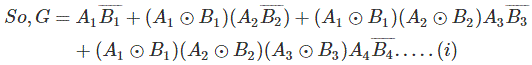Similarly,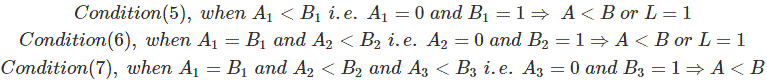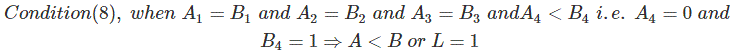Now,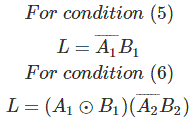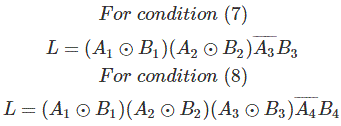Again when,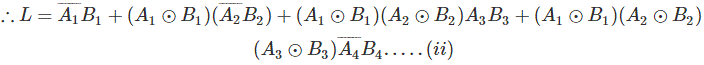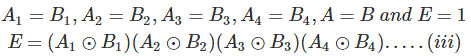The logic circuit can be drawn from the above equations (i), (ii) and (iii).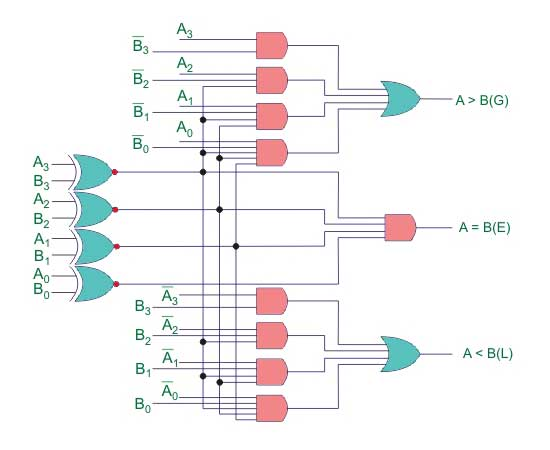This is 4bit digital comparator.

## IC of Digital Comparator

The Integrated Circuit (IC) available for 4 bit digital comparator is IC 7485. For more bit comparison, more than one such ICs can be cascaded. This IC has three terminals, labeled as (A < B)in, (A = B)in and (A > B)in and other three terminals labeled as, as (A < B)out, (A = B)out and (A > B)out. During cascading of two 7485 ICs, (A < B)out, (A = B)out and (A > B)out of lower order IC would be connected to (A < B)in, (A = B)in and (A > B)in of higher order IC, respectively.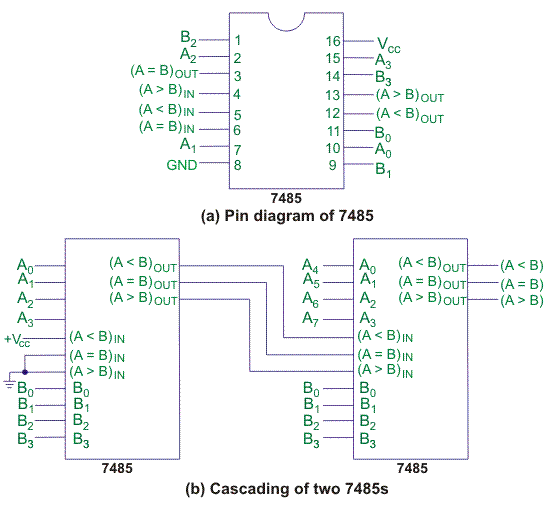Want To Learn Faster? 🎓
Get electrical articles delivered to your inbox every week.
No credit card required—it’s 100% free.